In the bottom of that sidebar, you find pictures and videos of my beautiful Colombia! Enjoy it!

Sudoku!

This is one of my hobbies. I share with you becouse is a good way to distract you of stress from University or School! I hope you like it! Oh, I'll give to you a little advice: When you finish it, please go back to study numerical methods.Sunday, May 16, 2010

*Bracketing methods

.
*Graphical Methods
-A simple method for obtaining an estimate of the root of the equation f(x)=0 is to make a plot of the function and observe where it crosses the x axis. This point wich represents the x value for wich
f(x)=0, provides a rough approximation of the root
.
Graphical techniques are of limited practical value because they are not precise. However, graphical methods can be utilized to obtain rough estimates of roots. These estimates can be employed as starting guesses for numerical methods.

Aside from providing rough estimates of the root, graphical interpretations are important tant tools understanding the properties of the functions and anticipating the pirfalls of the numerical methods.
If f(a) = f(b) ----> There are roots or a pair number of roots
If f(a) = f(b) ----> There is one root or an odd number of roots

Source: chapra fifth edition

Types of Methods

.

• Graphic Methods
• Bracketing Methods
• Open MethodsGraphic Methods

• No precise load
• Limited Value
• To estimate initial values
• Can prevent failures in the methods (asymptotes)
• Can be considered finite / conditional

Bracketing Methods : Bisection method, The false position method

• Guarantee convergence
• Begins by asking a range (which contient root)

Bisection Method

Search the "cota"(1) and cut the interval by the half

>Binary cut

>Partition

>Bolzano

Types of search

1. Define an interval in which at least has a root. The sign change method
2. Evaluated the image of the function in the "cotas"(1). The product of these images is below 0

f (a) . f (b) below 0

(If we do not know that there are more "cotas"(1) assume at least 1)

3. Interval is divided in half and is checked, assuming the value on the half is the root.

If f(a)*f(r) below 0

*Repeat as many times as tolerance tells me

4. Tolerance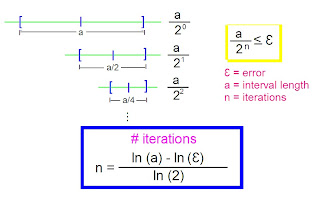Advantages
• All the bracketing methods converge
• Easy programming
• Has a very clear error handling

• Convergence may take a long
• Does not take into account extreme values ("cotas") as possible roots.

****(1) What I mean when I write "cota"?Sunday, May 09, 2010

*Taylor Theorem

.
Truncation error results from using an approximation in place of an exact mathematical procedure.
Non-elementary functions such as trigonometric, exponential, and others are expressed in an approximate fashion using Taylor series when their values, derivatives, and integrals are computed.
Any smooth function can be approximated as a polynomial. Taylor series provides a means to predict the value of a function at one point in terms of the function value and its derivatives at another point.

*Taylor Theorem

If the function f and its first n+1 derivatives are continuous on an interval containing a & x, then the value of x is given by•Any smooth function can be approximated as a polynomial.

f(xi+1) ≈ f(xi) zero order approximation, only true if xi+1 and xi are very close to each other.

f(xi+1) ≈ f(xi) + f′(xi) (xi+1-xi) first order approximation, in form of a straight line

nth order approximation: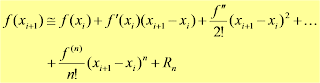(xi+1-xi)= h step size (define first)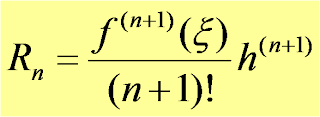Reminder term, Rn, accounts for all terms from (n+1) to infinity.

• x is not known exactly, lies somewhere between xi+1>x >xi .
• Need to determine f ^(n+1)(x), to do this you need f'(x).
• If we knew f(x), there wouldn’t be any need to perform the Taylor series expansion.
• However, R=O(h^(n+1)), (n+1)th order, the order of truncation error is hn+1.
• O(h), halving the step size will halve the error.
• O(h2), halving the step size will quarter the error.
• Truncation error is decreased by addition of terms to the Taylor series.
• If h is sufficiently small, only a few terms may be required to obtain an approximation close enough to the actual value for practical purposes.

•Numerical Differentiation: Finite Divided Differences
•Forward Difference
•Backward Difference
•Centered Difference

Error Definitions

.
Numerical errors arise from the use of approximation to represent exact mathematical operations and quantities. These include truncation errors, wich result when approximations are used to represent exact mathematical procedures, and round-off errors, wich result when the numbers having limited significant figures are used to represent exact numbers. For both types, the relationship between the exact, or true, result and the approximation can be formulated as

True value = approximation + error (1)

By rearranging (1), we find that numerical error is equal to the discrepancy between the truth and the approximation, as in

Et = true value - approximation (2)

where Et is used to designate the exact value of the error. The subscrit t is included to designate that this is the "true" error. This is contrast to other cases, as described shortly, where an "approximte" estimate of the error must be employed. A shortcoming of this definition is that it takes no account of the order of magnitude of the valued under examination. One wa to account for the magnitudes of the quantities being evaluated is to normalize the error to the true value, as inTrue fractional relative error = true error / true value (3)

where, as specified by (3), error = true value - approximation. The relative error can also be multiplied by 100 percent to express it as.

et = (true error / true value) * 100

where et designates the true percent relative error.

*Accuracy and Precision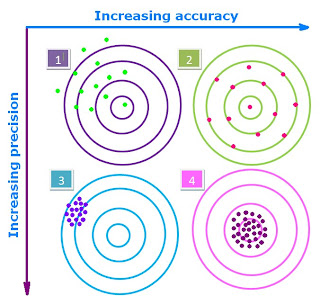The errors associated with both calculations and measurements can be characterized with regard to their accuracy and precision. Accuracy refers to how closely a computer or measured value agrees with the true value. Precision refers to how closely individual computed or measured values agree with each other. These concepts can be illustrated graphically ussing an analogy from target practice. The bullet holes on each target in Figure can be thought of as the predictions of a numerical technique, whereas the bull's-eye represents the truth. Inaccuracy is defined as systematic deviation from the truth. Thus, although the shots in Fig. (3) are more tightly grouped than those in Fig. (1), the two cases are equally biased because they are both centered on the upper left quadrant of the target. Imprecision, on the other hand, refers to the magnitude of the scatter. Therefore, although Fig. (2) and (4) are equaly accurate (that is, centered on the bull's-eye), the later is more precise because the shots are tightly grouped. Numerical methods should be sufficiently accurate or unbiased to meet the requirements of a particular engineering problem. They also should be precise enough for adequate engineering desing.

*Significant Figures

The significant figures of a number are those digits that carry meaning contributing to its precision (see entry for Accuracy and precision). This includes all digits except:
• leading and trailing zeros where they serve merely as placeholders to indicate the scale of the number.
• spurious digits introduced, for example, by calculations carried out to greater accuracy than that of the original data, or measurements reported to a greater precision than the equipment supports.

The concept of significant figures is often used in connection with rounding. Rounding to n significant figures is a more general-purpose technique than rounding to n decimal places, since it handles numbers of different scales in a uniform way. For example, the population of a city might only be known to the nearest thousand and be stated as 52,000, while the population of a country might only be known to the nearest million and be stated as 52,000,000. The former might be in error by hundreds, and the latter might be in error by hundreds of thousands, but both have two significant figures (5 and 2). This reflects the fact that the significance of the error (its likely size relative to the size of the quantity being measured) is the same in both cases.

Computer representations of floating point numbers typically use a form of rounding to significant figures, but with binary numbers.

The term "significant figures" can also refer to a crude form of error representation based around significant figure rounding; for this use, see Significance arithmetic.

*Approximations and Round-Off Errors

For many applied engineering problems, we cannot obtain analytical solutions. Therefore, we cannot compute exactly the errors associated with our numerical methods. In these case, we must settle for approximations or estimates of the errors.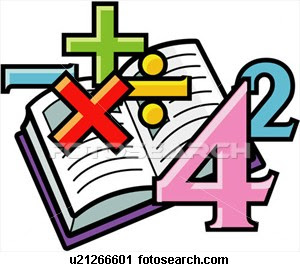Such errors are characteristic of most of the techniques. In professional practice, errors can be costly and sometimes catastrophic. If a structure or device fails, lives can be lost. On of the two major forms of numerical error: round-off error and truncation error.

Round-off error

Is due to the fact that computers can represent only quantities with a finite number of digits.

Truncation Error

Is the discrepancy introduced by the fact that numerical methods may employ approximations to represent exact mathematical operations and quatities.

*Mathematical Model*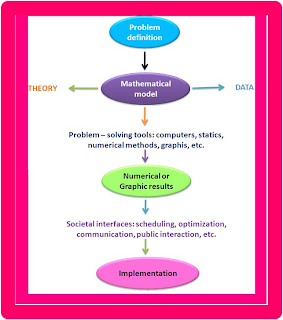A mathematical model can be broadly defined as a formulation or equation that expresses the essential features of a physical system or process in mathematical terms. In a very general sense, it can be represented as a functional relationship of the form

Dependent variable = f (independent variables, parameters, forcing functions)

(Eq (1))

Fig. The engineering problem: solving process.)

where the dependent variable is a characteristic that usually reflects the behavior or state of the system; the independent variable are usually dimensions, such as time and space, along wich the system's behavior is being determined; the parameters are reflective of the system's properties or composition; and the forcing functions are external influences acting upon the system.The actual mathematical expression of Eq. (1) can range from a simple algebraic relationship to large complicated sets of differential equations. For example, on the basis of his observations, Newton formulated his second law of motion, which states that the time rate of change of momentum of a body is equal to the resultant force acting on it. The mathematical expression, or model,

of the second law is the well-known equation

F = m.a (Eq (2))

where F = net force acting on the body, m = mass of the object, and a = its acceleration.

The second law can be recast in the format of Eq (1) by merely dividing both sides by m to giveCharacteristics that are typical of mathematical models of the physical world:

1. It describes a natural process or system in mathematical terms.

2. It represents an idealization and simplification of reality. That is, the model ignores negligible details of the natural process and focuses on its essential manifestations. Thus, the second law does not include the effects of relativity that are minimal importance when applied to objects and forces that interact on or about the earth's surface at velocities and on scales visible to humans.

3. Finally, it yields reproducible results and, consequently, can be used for predictive purposes.This equation, is called an analytical, or exact, solution because it exactly satisfies the original differential equation. Unfortunately, there are many mathematical models that cannot be solved exactly. In many of these cases, the only alternative is to develop a numerical solution that approximates the exact solution.

As conclution, numerical methods are those in wich the matematical problem is reformulated so it can be solved by arithmetic operations.

Source:
Numerical methods for Engineers
Fifth Edition
Steven C. Chapra
Raymond P. Canale
Mc Graw Hill

Friday, May 07, 2010

Model TypesSource:
Métodos numéricos en ingeniería/
Capítulo I/Elkin Santafe/

Elementos básicos de modelamiento matemático

Monday, May 03, 2010

Welcome!! :)Welcome to Numerical Methods Blog!

This is a space for you!On these days I will upload some contents about numerical methods. Wait for them! Ask what you want, I will try to answer to you.